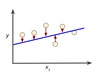loss function

• ## 损失函数

千次阅读 2018-04-28 20:08:37
损失函数介绍损失函数用于描述模型预测值与真实值的差距大小。一般有有两种常见的算法——均值平方差（MSE）和交叉熵。下面来分别介绍每个算法的具体内容。1 均值平方差均值平方差（Mean Squared Error，MSE），...
一 损失函数介绍损失函数用于描述模型预测值与真实值的差距大小。一般有有两种常见的算法——均值平方差（MSE）和交叉熵。下面来分别介绍每个算法的具体内容。1 均值平方差均值平方差（Mean Squared Error，MSE），也称“均方误差”，在神经网络中主要是表达预测值和真实值之间的差异，在数理统计中，均方误差是指参数估计值与参数真值之差平方的预期值。公式如下：主要是对每一个真实值与预期值相减的平方取平均值：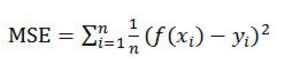均方误差的值越小，表明模型越好。类似的损失算法还有均方根误差RMSE（将MSE开平方）：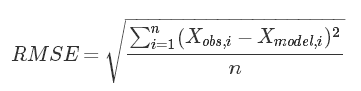平均绝对值误差MAD（对一个真实值与预测值相减的绝对值取平均值）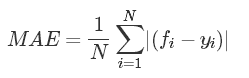2 交叉熵交叉熵（crossentropy）也是loss算法的一种，一般用在分类问题上，表达意思为预测输入样本属于哪一类的概率。其表达式如下，其中y代表真实值分类（0或1），a代表预测值。二分类情况下的公式：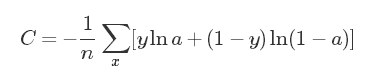交叉熵也是值越小，代表预测结果越准。3 损失算法的选取损失函数的选取取决于输入标签数据的类型：如果输入的实数、无界的值，损失函数使用平方差。如果输入标签是位矢量（分类标志），使用交叉熵会更适合。二 损失函数举例下面是3个训练样本经过使用softmax作为激活函数的神经网络的输出(computed)、真实结果(targets)以及是否预测正确的对比表格。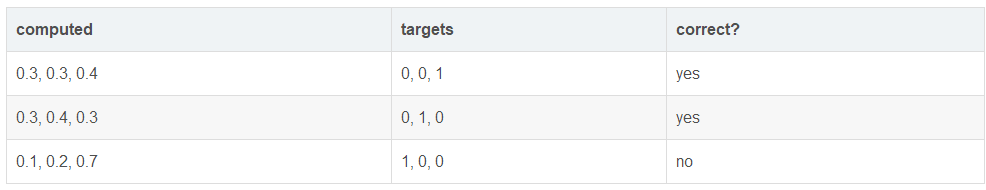下面是另外一个网络的训练结果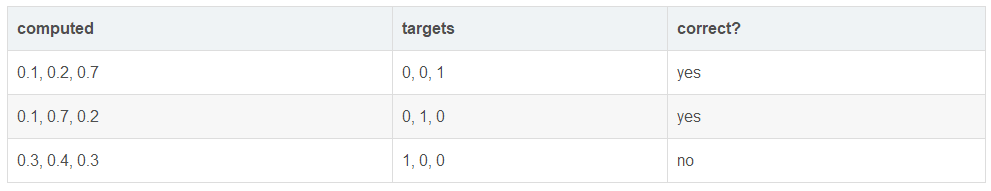1 交叉熵计算（三分类交叉熵）第一个样本的交叉熵为：−(0∗ln0.3+0∗ln0.3+1∗ln0.4)=−ln0.4对于神经网络来说，交叉熵的计算有点古怪，因为只有一项会被保留下来。因此三个样本的平均交叉熵为：−(ln0.4+ln0.4+ln0.1)/3=1.38第二个网络的平均交叉熵为：−(ln0.7+ln0.7+ln0.3)/3=0.642 均方误差(Root Mean Square Error, RMSE)第一个网络：0.81第二个网络：0.34三 参考https://blog.csdn.net/u014755493/article/details/71402991https://blog.csdn.net/DawnRanger/article/details/78031793
展开全文• 有监督学习机器学习分为有监督学习，无监督学习，半监督学习，强化学习。对于逻辑回归来说，就是一种典型的有监督学习。 既然是有监督学习，训练集自然可以用如下方式表述： {(x1,y1),(x2,y2),⋯,(xm,ym)}\{(x^1,y...
项目github地址：bitcarmanlee easy-algorithm-interview-and-practice
欢迎大家star，留言，一起学习进步
有监督学习
机器学习分为有监督学习，无监督学习，半监督学习，强化学习。对于逻辑回归来说，就是一种典型的有监督学习。
既然是有监督学习，训练集自然可以用如下方式表述：{(x1,y1),(x2,y2),⋯ ,(xm,ym)}\{(x^1,y^1),(x^2,y^2),\cdots,(x^m,y^m)\}
对于这m个训练样本，每个样本本身有n维特征。再加上一个偏置项x0x_0, 则每个样本包含n+1维特征：x=[x0,x1,x2,⋯ ,xn]Tx = [x_0,x_1,x_2,\cdots,x_n]^T
其中 x∈Rn+1x\in R^{n+1}, x0=1x_0=1, y∈{0,1}y\in\{0,1\}
李航博士在统计学习方法一书中给分类问题做了如下定义：
分类是监督学习的一个核心问题，在监督学习中，当输出变量Y取有限个离散值时，预测问题便成为分类问题。这时，输入变量X可以是离散的，也可以是连续的。监督学习从数据中学习一个分类模型或分类决策函数，称为分类器(classifier)。分类器对新的输入进行输出的预测(prediction)，称为分类(classification).
在logistic回归详解一(http://blog.csdn.net/bitcarmanlee/article/details/51154481）中，我们花了一整篇篇幅阐述了为什么要使用logistic函数: hθ(x)=g(θTx)=11+e−θTxh_{\theta}(x) = g(\theta^{T}x) = \frac{1}{1+e^{-\theta^{T}x}}
其中一个重要的原因，就是要将Hypothesis(NG课程里的说法)的输出映射到0与1之间，既：0≤hθ(x)≤10\le h_{\theta}(x)\le 1
同样是李航博士统计学习方法一书中，有以下描述：
统计学习方法都是由模型，策略，和算法构成的，即统计学习方法由三要素构成，可以简单表示为：方法=模型+策略+算法方法 = 模型 + 策略 + 算法
对于logistic回归来说，模型自然就是logistic回归，策略最常用的方法是用一个损失函数(loss function)或代价函数(cost function)来度量预测错误程度，算法则是求解过程，后期会详细描述相关的优化算法。
logistic函数求导
KaTeX parse error: No such environment: align at position 7: \begin{̲a̲l̲i̲g̲n̲}̲
g'(z) & = \fra…
此求导公式在后续推导中会使用到
常见的损失函数
机器学习或者统计机器学习常见的损失函数如下：
1.0-1损失函数 （0-1 loss function）L(Y,f(X))={1,Y ≠ f(X)0,Y = f(X) L(Y,f(X))= \begin{cases} 1 , & \text {Y $\neq$ f(X)} \\ 0, & \text{Y = f(X)} \end{cases}
2.平方损失函数（quadratic loss function)L(Y,f(X))=(Y−f(x))2L(Y,f(X)) = (Y - f(x))^2
3.绝对值损失函数(absolute loss function)L(Y,f(x))=∣Y−f(X)∣L(Y,f(x)) = |Y - f(X)|
4.对数损失函数（logarithmic loss function) 或对数似然损失函数(log-likehood loss function)L(Y,P(Y∣X))=−logP(Y∣X)L(Y,P(Y|X)) = -logP(Y|X)
逻辑回归中，采用的则是对数损失函数。如果损失函数越小，表示模型越好。
说说对数损失函数与平方损失函数
在逻辑回归的推导中国，我们假设样本是服从伯努利分布(0-1分布)的，然后求得满足该分布的似然函数，最终求该似然函数的极大值。整体的思想就是求极大似然函数的思想。而取对数，只是为了方便我们的在求MLE(Maximum Likelihood Estimation)过程中采取的一种数学手段而已。
损失函数详解
根据上面的内容，我们可以得到逻辑回归的对数似然损失函数cost function：cost(hθ(x),y)={−log(hθ(x))if y=1−log(1−hθ(x))if y=0cost(h_{\theta}(x),y) = \begin{cases}  -log(h_{\theta}(x))  & \text {if y=1} \\ -log(1-h_{\theta}(x))  & \text{if y=0} \end{cases}
稍微解释下这个损失函数，或者说解释下对数似然损失函数：
当y=1时，假定这个样本为正类。如果此时hθ(x)=1h_\theta(x)=1,则单对这个样本而言的cost=0,表示这个样本的预测完全准确。那如果所有样本都预测准确，总的cost=0
但是如果此时预测的概率hθ(x)=0h_\theta(x)=0，那么cost→∞cost\to\infty。直观解释的话，由于此时样本为一个正样本，但是预测的结果P(y=1∣x;θ)=0P(y=1|x;\theta) = 0, 也就是说预测 y=1的概率为0，那么此时就要对损失函数加一个很大的惩罚项。
当y=0时，推理过程跟上述完全一致，不再累赘。
将以上两个表达式合并为一个，则单个样本的损失函数可以描述为：cost(hθ(x),y)=−yilog(hθ(x))−(1−yi)log(1−hθ(x))cost(h_{\theta}(x),y) = -y_ilog(h_{\theta}(x)) - (1-y_i)log(1-h_{\theta}(x))
因为 yiy_i 只有两种取值情况，1或0，分别令y=1或y=0，即可得到原来的分段表示式。
全体样本的损失函数可以表示为：cost(hθ(x),y)=∑i=1m−yilog(hθ(x))−(1−yi)log(1−hθ(x))cost(h_{\theta}(x),y) = \sum_{i=1}^{m} -y_ilog(h_{\theta}(x)) - (1-y_i)log(1-h_{\theta}(x))
这就是逻辑回归最终的损失函数表达式


展开全文logistic回归 详解
• ## 交叉熵损失函数原理详解

万次阅读 多人点赞 2019-08-30 10:28:42
之前在代码中经常看见交叉熵损失函数(CrossEntropy Loss)，只知道它是分类问题中经常使用的一种损失函数，对于其内部的原理总是模模糊糊，而且一般使用交叉熵作为损失函数时，在模型的输出层总会接一个softmax函数，...
交叉熵损失函数原理详解
之前在代码中经常看见交叉熵损失函数(CrossEntropy Loss)，只知道它是分类问题中经常使用的一种损失函数，对于其内部的原理总是模模糊糊，而且一般使用交叉熵作为损失函数时，在模型的输出层总会接一个softmax函数，至于为什么要怎么做也是不懂，所以专门花了一些时间打算从原理入手，搞懂它，故在此写一篇博客进行总结，以便以后翻阅。

交叉熵简介
交叉熵是信息论中的一个重要概念，主要用于度量两个概率分布间的差异性，要理解交叉熵，需要先了解下面几个概念。

信息量
信息奠基人香农（Shannon）认为“信息是用来消除随机不确定性的东西”，也就是说衡量信息量的大小就是看这个信息消除不确定性的程度。
“太阳从东边升起”，这条信息并没有减少不确定性，因为太阳肯定是从东边升起的，这是一句废话，信息量为0。
”2018年中国队成功进入世界杯“，从直觉上来看，这句话具有很大的信息量。因为中国队进入世界杯的不确定性因素很大，而这句话消除了进入世界杯的不确定性，所以按照定义，这句话的信息量很大。
根据上述可总结如下：信息量的大小与信息发生的概率成反比。概率越大，信息量越小。概率越小，信息量越大。
设某一事件发生的概率为P(x)，其信息量表示为：
$I\left ( x \right ) = -\log\left ( P\left ( x \right ) \right )$
其中$I\left ( x \right )$表示信息量，这里$\log$表示以e为底的自然对数。

信息熵
信息熵也被称为熵，用来表示所有信息量的期望。
期望是试验中每次可能结果的概率乘以其结果的总和。
所以信息量的熵可表示为：（这里的$X$是一个离散型随机变量）
$H\left ( \mathbf{X} \right ) = -\sum \limits_{i=1}^n P(x_{i}) \log \left ( P \left ( x_{i} \right ))) \qquad ( \mathbf{X}= x_{1},x_{2},x_{3}...,x_{n} \right)$
使用明天的天气概率来计算其信息熵：

序号
事件
概率P
信息量

1
明天是晴天
0.5
$-\log \left ( 0.5 \right )$

2
明天出雨天
0.2
$-\log \left ( 0.2 \right )$

3
多云
0.3
$-\log \left ( 0.3 \right )$

$H\left ( \mathbf{X} \right ) = -\left ( 0.5 * \log \left ( 0.5 \right ) + 0.2 * \log \left ( 0.2 \right ) + 0.3 * \log \left ( 0.3 \right ) \right)$
对于0-1分布的问题，由于其结果只用两种情况，是或不是，设某一件事情发生的概率为$P\left ( x \right )$，则另一件事情发生的概率为$1-P\left ( x \right )$，所以对于0-1分布的问题，计算熵的公式可以简化如下：
$H\left ( \mathbf{X} \right ) = -\sum \limits_{n=1}^n P(x_{i}\log \left ( P \left ( x_{i} \right )) \right) \\ = -\left [ P\left ( x \right) \log \left ( P\left ( x \right ) \right ) + \left ( 1 - P\left ( x \right ) \right) \log \left ( 1-P\left ( x \right ) \right ) \right] \\ = -P\left ( x \right) \log \left ( P\left ( x \right ) \right ) - \left ( 1 - P\left ( x \right ) \right) \log \left ( 1-P\left ( x \right ) \right)$

相对熵（KL散度）
如果对于同一个随机变量$X$有两个单独的概率分布$P\left(x\right)$和$Q\left(x\right)$，则我们可以使用KL散度来衡量这两个概率分布之间的差异。
下面直接列出公式，再举例子加以说明。
$D_{KL}\left ( p || q \right) = \sum \limits_{i=1}^n p\left ( x_{i}\right ) \log \left ( \frac{p\left ( x_{i} \right )}{q\left ( x_{i} \right )} \right )$
在机器学习中，常常使用$P\left(x\right)$来表示样本的真实分布，$Q \left(x\right)$来表示模型所预测的分布，比如在一个三分类任务中（例如，猫狗马分类器），$x_{1}, x_{2}, x_{3}$分别代表猫，狗，马，例如一张猫的图片真实分布$P\left(X\right) = [1, 0, 0]$, 预测分布$Q\left(X\right) = [0.7, 0.2, 0.1]$，计算KL散度：
$D_{KL}\left ( p || q \right) = \sum \limits_{i=1}^n p\left ( x_{i}\right ) \log \left ( \frac{p\left ( x_{i} \right )}{q\left ( x_{i} \right )} \right ) \\ = p\left ( x_{1}\right ) \log \left ( \frac{p\left ( x_{1} \right )}{q\left ( x_{1} \right )} \right ) + p\left ( x_{2}\right ) \log \left ( \frac{p\left ( x_{2} \right )}{q\left ( x_{2} \right )} \right ) + p\left ( x_{3}\right ) \log \left ( \frac{p\left ( x_{3} \right )}{q\left ( x_{3} \right )} \right ) \\ = 1 * \log \left ( \frac{1}{0.7} \right ) = 0.36$
KL散度越小，表示$P\left(x\right)$与$Q\left(x\right)$的分布更加接近，可以通过反复训练$Q\left(x \right)$来使$Q\left(x \right)$的分布逼近$P\left(x \right)$。

交叉熵
首先将KL散度公式拆开：
$D_{KL}\left ( p || q \right) = \sum \limits_{i=1}^n p\left ( x_{i}\right ) \log \left ( \frac{p\left ( x_{i} \right )}{q\left ( x_{i} \right )} \right ) \\ = \sum \limits_{i=1}^n p \left (x_{i}\right) log \left(p \left (x_{i}\right)\right) - \sum \limits_{i=1}^n p \left (x_{i}\right) log \left(q \left (x_{i}\right)\right) \\ = -H \left (p \left(x \right) \right) + \left [-\sum \limits_{i=1}^n p \left (x_{i}\right) log \left(q \left (x_{i}\right)\right) \right]$
前者$H \left (p \left (x \right)\right)$表示信息熵，后者即为交叉熵，KL散度 = 交叉熵 - 信息熵
交叉熵公式表示为：
$H \left (p, q\right) = -\sum \limits_{i=1}^n p \left (x_{i}\right) log \left(q \left (x_{i}\right)\right)$
在机器学习训练网络时，输入数据与标签常常已经确定，那么真实概率分布$P\left(x \right)$也就确定下来了，所以信息熵在这里就是一个常量。由于KL散度的值表示真实概率分布$P\left(x\right)$与预测概率分布$Q \left(x\right)$之间的差异，值越小表示预测的结果越好，所以需要最小化KL散度，而交叉熵等于KL散度加上一个常量（信息熵），且公式相比KL散度更加容易计算，所以在机器学习中常常使用交叉熵损失函数来计算loss就行了。

交叉熵在单分类问题中的应用
在线性回归问题中，常常使用MSE(Mean Squared Error)作为loss函数，而在分类问题中常常使用交叉熵作为loss函数。
下面通过一个例子来说明如何计算交叉熵损失值。
假设我们输入一张狗的图片，标签与预测值如下：

*
猫
狗
马

Label
0
1
0

Pred
0.2
0.7
0.1

那么loss
$loss = -\left ( 0 * \log \left ( 0.2 \right ) + 1 * \log \left ( 0.7 \right ) + 0 * \log \left ( 0.1 \right )\right) = 0.36$
一个batch的loss为
$loss = -\frac{1}{m}\sum \limits_{i=1}^m \sum \limits_{j=1}^n p \left (x_{ij}\right) log \left(q \left (x_{ij}\right)\right)$
其中m表示样本个数。

总结：

交叉熵能够衡量同一个随机变量中的两个不同概率分布的差异程度，在机器学习中就表示为真实概率分布与预测概率分布之间的差异。交叉熵的值越小，模型预测效果就越好。

交叉熵在分类问题中常常与softmax是标配，softmax将输出的结果进行处理，使其多个分类的预测值和为1，再通过交叉熵来计算损失。

参考：
https://blog.csdn.net/tsyccnh/article/details/79163834

THE END展开全文分类 机器学习
• 说起交叉熵损失函数「Cross Entropy Loss」，脑海中立马浮现出它的公式： 我们已经对这个交叉熵函数非常熟悉，大多数情况下都是直接拿来使用就好。但是它是怎么来的？为什么它能表征真实样本标签和预测概率之间的...
说起交叉熵损失函数「Cross Entropy Loss」，脑海中立马浮现出它的公式：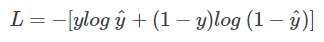我们已经对这个交叉熵函数非常熟悉，大多数情况下都是直接拿来使用就好。但是它是怎么来的？为什么它能表征真实样本标签和预测概率之间的差值？上面的交叉熵函数是否有其它变种？

1.交叉熵损失函数的推导

我们知道，在二分类问题模型：例如逻辑回归「Logistic Regression」、神经网络「Neural Network」等，真实样本的标签为 [0，1]，分别表示负类和正类。模型的最后通常会经过一个 Sigmoid 函数，输出一个概率值，这个概率值反映了预测为正类的可能性：概率越大，可能性越大。
Sigmoid 函数的表达式和图形如下所示：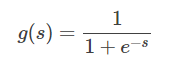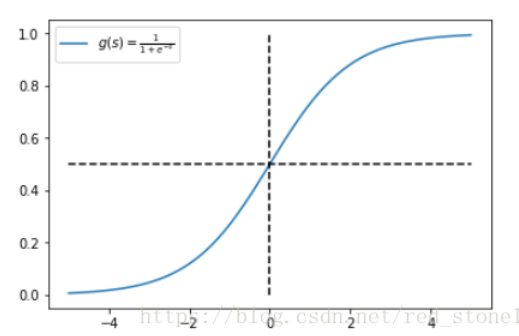其中 s 是模型上一层的输出，Sigmoid 函数有这样的特点：s = 0 时，g(s) = 0.5；s >> 0 时， g ≈ 1，s << 0 时，g ≈ 0。显然，g(s) 将前一级的线性输出映射到 [0，1] 之间的数值概率上。这里的 g(s) 就是交叉熵公式中的模型预测输出 。

我们说了，预测输出即 Sigmoid 函数的输出表征了当前样本标签为 1 的概率：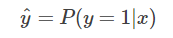很明显，当前样本标签为 0 的概率就可以表达成：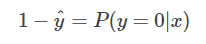重点来了，如果我们从极大似然性的角度出发，把上面两种情况整合到一起：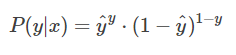不懂极大似然估计也没关系。我们可以这么来看：

当真实样本标签 y = 0 时，上面式子第一项就为 1，概率等式转化为：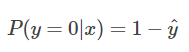当真实样本标签 y = 1 时，上面式子第二项就为 1，概率等式转化为：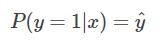两种情况下概率表达式跟之前的完全一致，只不过我们把两种情况整合在一起了。

重点看一下整合之后的概率表达式，我们希望的是概率 P(y|x) 越大越好。首先，我们对 P(y|x) 引入 log 函数，因为 log 运算并不会影响函数本身的单调性。则有：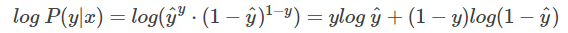我们希望 log P(y|x) 越大越好，反过来，只要 log P(y|x) 的负值 -log P(y|x) 越小就行了。那我们就可以引入损失函数，且令 Loss = -log P(y|x)即可。则得到损失函数为：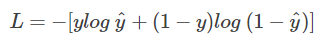非常简单，我们已经推导出了单个样本的损失函数，是如果是计算 N 个样本的总的损失函数，只要将 N 个 Loss 叠加起来就可以了：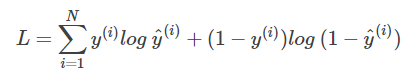这样，我们已经完整地实现了交叉熵损失函数的推导过程。

2. 交叉熵损失函数的直观理解

我已经知道了交叉熵损失函数的推导过程。但是能不能从更直观的角度去理解这个表达式呢？而不是仅仅记住这个公式。好问题！接下来，我们从图形的角度，分析交叉熵函数，加深理解。

首先，还是写出单个样本的交叉熵损失函数：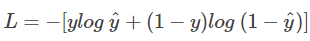我们知道，当 y = 1 时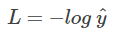这时候，L 与预测输出的关系如下图所示：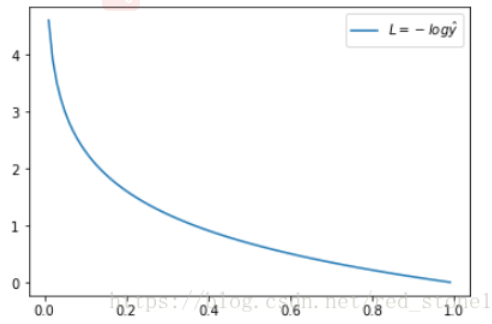看了 L 的图形，简单明了！横坐标是预测输出，纵坐标是交叉熵损失函数 L。显然，预测输出越接近真实样本标签 1，损失函数 L 越小；预测输出越接近 0，L 越大。因此，函数的变化趋势完全符合实际需要的情况。

当 y = 0 时：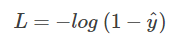这时候，L 与预测输出的关系如下图所示：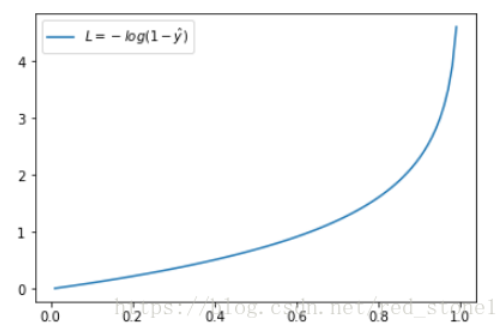同样，预测输出越接近真实样本标签 0，损失函数 L 越小；预测函数越接近 1，L 越大。函数的变化趋势也完全符合实际需要的情况。

从上面两种图，可以帮助我们对交叉熵损失函数有更直观的理解。无论真实样本标签 y 是 0 还是 1，L 都表征了预测输出与 y 的差距。

另外，重点提一点的是，从图形中我们可以发现：预测输出与 y 差得越多，L 的值越大，也就是说对当前模型的 “ 惩罚 ” 越大，而且是非线性增大，是一种类似指数增长的级别。这是由 log 函数本身的特性所决定的。这样的好处是模型会倾向于让预测输出更接近真实样本标签 y。

3. 交叉熵损失函数的其它形式

什么？交叉熵损失函数还有其它形式？没错！我刚才介绍的是一个典型的形式。接下来我将从另一个角度推导新的交叉熵损失函数。

这种形式下假设真实样本的标签为 +1 和 -1，分别表示正类和负类。有个已知的知识点是Sigmoid 函数具有如下性质：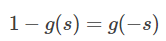这个性质我们先放在这，待会有用。

好了，我们之前说了 y = +1 时，下列等式成立：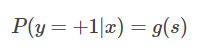如果 y = -1 时，并引入 Sigmoid 函数的性质，下列等式成立：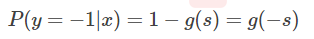重点来了，因为 y 取值为 +1 或 -1，可以把 y 值带入，将上面两个式子整合到一起：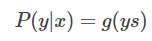这个比较好理解，分别令 y = +1 和 y = -1 就能得到上面两个式子。

接下来，同样引入 log 函数，得到：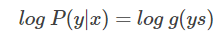要让概率最大，反过来，只要其负数最小即可。那么就可以定义相应的损失函数为：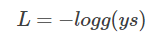还记得 Sigmoid 函数的表达式吧？将 g(ys) 带入：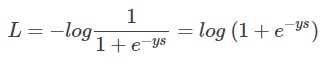好咯，L 就是我要推导的交叉熵损失函数。如果是 N 个样本，其交叉熵损失函数为：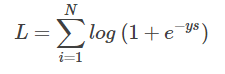接下来，我们从图形化直观角度来看。当 y = +1 时：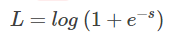这时候，L 与上一层得分函数 s 的关系如下图所示：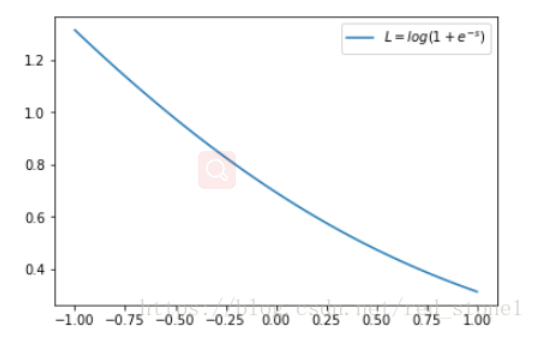横坐标是 s，纵坐标是 L。显然，s 越接近正无穷，损失函数 L 越小；s 越接近负无穷，L 越大。

另一方面，当 y = -1 时：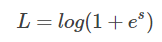这时候，L 与上一层得分函数 s 的关系如下图所示：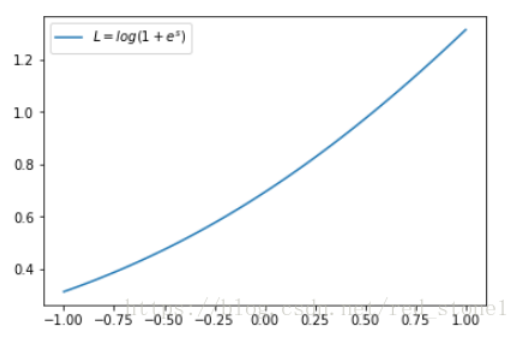同样，s 越接近负无穷，损失函数 L 越小；s 越接近正无穷，L 越大。

4.总结

本文主要介绍了交叉熵损失函数的数学原理和推导过程，也从不同角度介绍了交叉熵损失函数的两种形式。第一种形式在实际应用中更加常见，例如神经网络等复杂模型；第二种多用于简单的逻辑回归模型。

需要注意的是：第一个公式中的变量是sigmoid输出的值，第二个公式中的变量是sigmoid输入的值。
展开全文• 上图证明,权重的导数和误差有某种关系,计算的时候也会用到,也就是说误差可以通过某种函数表达权重导数,导数的反导就是这个函数 所以神经网络的loss函数非常重要, 直接影响神经网络的结构和物理意义,虽然我们无法理解...
• 交叉熵损失函数 交叉熵损失函数的求导 前言 说明：本文只讨论Logistic回归的交叉熵，对Softmax回归的交叉熵类似。 首先，我们二话不说，先放出交叉熵的公式： J(θ)=−1m∑i=1my(i)log(hθ(x(i)))+(1−y(i))...logistic回归 逻辑回归
• 来写一个softmax求导的推导过程，不仅可以给自己理清思路，还可以造福大众，岂不美哉~ softmax经常被添加在分类任务的...softmax(柔性最大值)函数，一般在神经网络中， softmax可以作为分类任务的输出层。其实可...神经网络
•pytorch
• 对于机器学习中常见的损失函数有：平方损失函数与交叉熵损失函数。在本文中将讲述两者含义与响应的运用区别。 2. 平方损失函数 平方损失函数较为容易理解，它直接测量机器学习模型的输出与实际结果之间的距离。...
• L1 和 L2 是机器学习中的两个损失函数，用于最小化误差。 L1 损失函数代表最小绝对偏差，也称为 LAD L2 损失函数代表最小二乘误差，也称为 LS 文章目录一、L1 损失函数二、L2 损失函数三、如何确定L1 和 L2 损失函数...
• 而centerloss的center是在loss.backward()之后对其进行修改，那么第二个损失函数也应该类似，也就是说在loss.backward()之前，模型输出不参与第二个损失函数的任何计算，只参与backward()之后更新center的步骤 ...深度学习 python
• 损失函数定义 损失函数（Loss function）是用来估量你模型的预测值 f(x)f(x) 与真实值 YY 的不一致程度，它是一个非负实值函数，通常用 L(Y,f(x))L(Y,f(x)) 来表示。损失函数越小，模型的鲁棒性就越好。损失函数是......